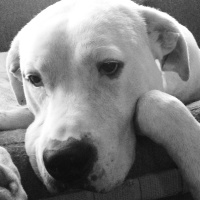wesen

Email

# Statistics One - Lab 3

### Lab goals:

• Read a datafile in R
• Print summary statistics
• Conduct correlational analyses
• Examine relationships among variables using scatterplots

### Example

• Investigating the effects of sport-related concussion

Reading in the data

### Correlation analysis of baseline measures

Round to 2 decimal places.

Create two subsets, control and concussedd

Correlation analysis of the control group, all measures. This is test/retest, so we can test different measures for reliability.

Let’s look at the concussed athletes. We can’t treat this data as test-retest, as there has been the concussion between the two measurements.

Does baseline impulse control predict memory impairment after a concussion?

### Scatterplots

Scatterplot functions are available in many packages and offer an array of advanced features. For the sake of time, I will demonstrate just a few examples here.

Standard scatterplot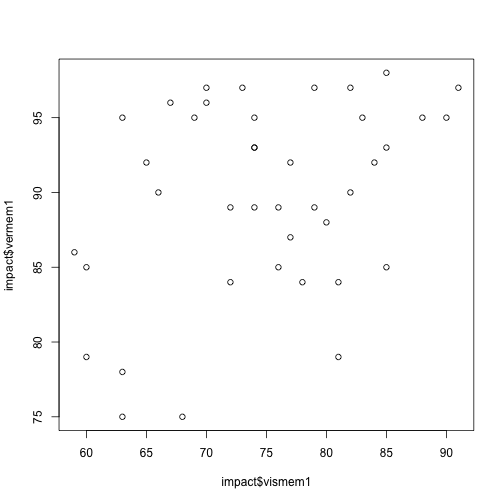Standard scatterplot with regression line. Positive slope means there is a positive correlation.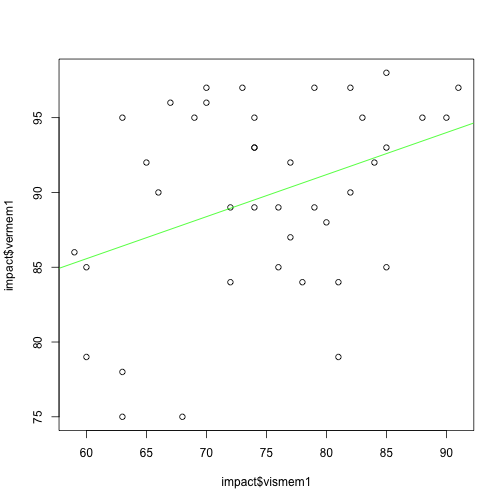Scatterplot matrix, in order to plot multiple correlations at once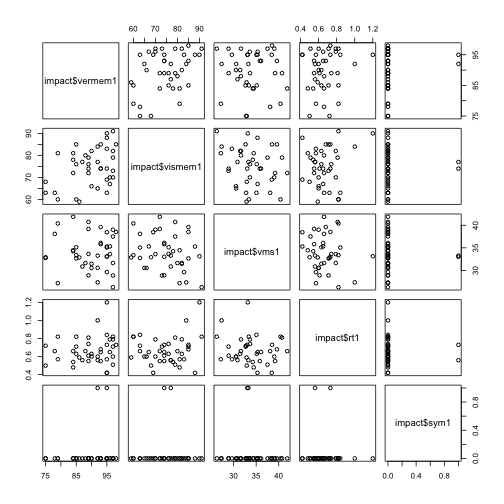Colored scatterplot matrix, ordered by magnitude of r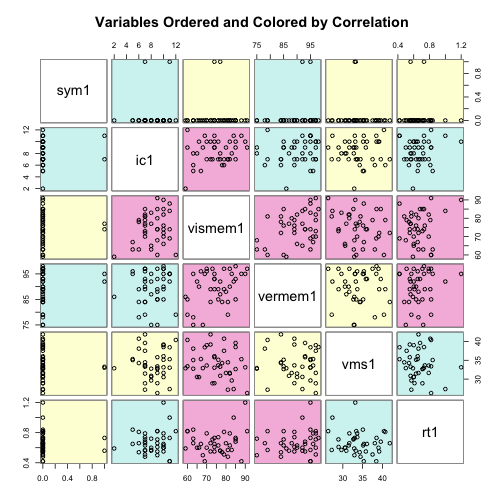Scatterplots in 3D